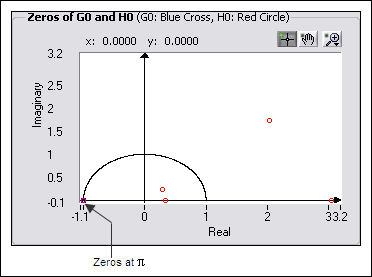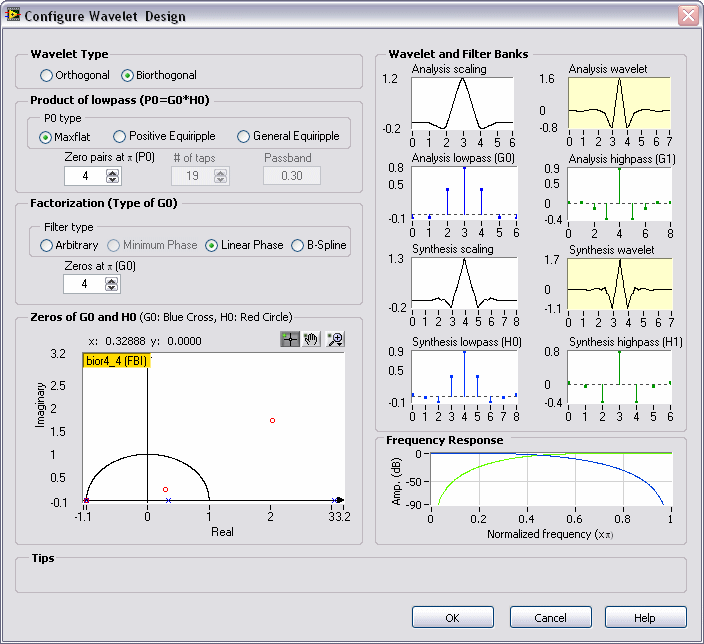# Example: Designing the FBI Wavelet (Advanced Signal Processing Toolkit)

LabVIEW 2014 Advanced Signal Processing Toolkit Help

Edition Date: June 2014

Part Number: 372656C-01

»View Product InfoDownload Help (Windows Only)

Different signal processing applications require different properties of wavelets. For image compression, you need a wavelet that is smooth, linear phase, and orthogonal. However, you cannot achieve all those properties simultaneously. One thing you can do is to ensure smoothness (higher order) and linear phase first and then pursue near-orthogonality.

Using the Wavelet Design Express VI, you can design a wavelet with specific properties. For example, you can complete the following steps to design the FBI wavelet, which is linear phase and near-orthogonal.

1. Add the Wavelet Design Express VI on the block diagram. The Configure Wavelet Design dialog box automatically launches.

2. Select Biorthogonal as the Wavelet Type because only biorthogonal wavelets have the linear-phase property.
3. In the Product of lowpass (G0*H0) section, select Maxflat as the P0 type and set the value of Zero pairs at(P0) to 4.

When you set parameters on the left-hand side of the configuration dialog box, plots of the designed wavelet and the associated filter banks interactively appear on the right-hand side.
4. In the Factorization (Type of G0) section, select Linear Phase as the Filter type and set the value of Zeros at(G0) to 4 because the wavelet must be near-orthogonal, meaning that G0(z) and H0(z) have the same or almost the same amount of zeros. By setting the value of Zeros at(G0) to 4, you can ensure that both G0(z) and H0(z) have the same amount of zeros at.

The following figure shows the zeros of G0(z) and H0(z).In the previous figure, notice that besides the four zeros assigned to H0(z) at π, the Zeros of G0 and H0 graph also contains six more zeros that belong to H0(z). Note that two zeros are on the negative half plane and do not appear on this graph.
5. To make the number of zeros of G0(z) close to that of H0(z), click either of the two zeros (o) of H0(z) near the bottom of the Zeros of G0 and H0 graph and switch the two zeros to those of G0(z). G0(z) now has six zeros and H0(z) has eight zeros.

The following figure shows the design result. Notice that the analysis and synthesis scaling functions are similar, and the analysis and synthesis wavelets also are similar, which means the designed wavelet is near-orthogonal. The symmetry of the filter banks also preserves the linear-phase property.Because of the near-orthogonality and linear-phase properties of the FBI wavelet, you can apply this wavelet to many kinds of signal and image processing, for example, image compression in JPEG2000. The FBI wavelet is called bior4_4 because both the analysis and synthesis lowpass filters G0(z) and H0(z) have four zeros at. This wavelet also is known as CDF 9, 7 because the lengths of the analysis and synthesis highpass filters are nine and seven, respectively.